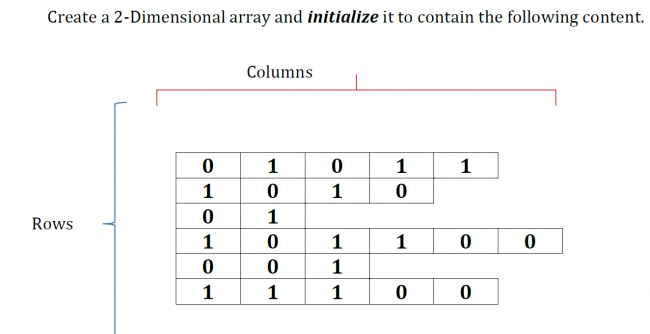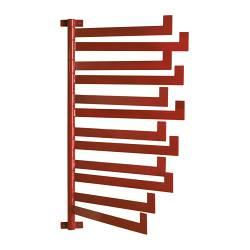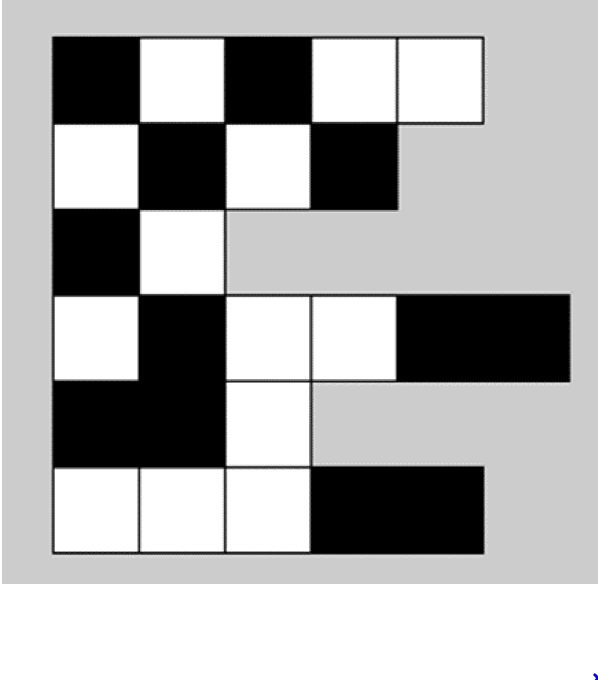#### Howdy, Stranger!

We are about to switch to a new forum software. Until then we have removed the registration on this forum.

# How would I make this 2D array?

edited November 2016

hey guys I'm supposed to make this 2D array and so far i've got the values in but the ends are being filled with zeroes. Here's the instructionsand here's my code:

``````    void setup()
{
int [][] arr= new int;
arr=0;
arr=1;
arr=0;
arr=1;
arr=1;
arr=1;
arr=0;
arr=1;
arr=0;
arr=0;
arr=1;
arr=1;
arr=0;
arr=1;
arr=1;
arr=0;
arr=0;
arr=0;
arr=0;
arr=1;
arr=1;
arr=1;
arr=1;
arr=0;
arr=0;

for (int i=0; i<arr.length; i++)
{
for (int j=0; j<arr.length; j++)
{
print(arr[i][j]);
}
println();
}
}
``````
Tagged:

• Which indexes are different from what you expect? Which lines are you initializing those indexes on?

If I were you, I would get out a piece of paper and a pencil. Draw a grid that shows the indexes and the value you want in that index in each cell. Then look at your code and see where you're setting those indexes.

• @ENGG233 --

"but the ends are being filled with zeroes"

Your `new int` will be made of ints (6x6 of them), and ints each have a default value of 0 -- see the Default Values section of Java datatypes.

Then you explicitly make some of them 0s and 1s. The rest are still 0s.

So either:

2. Your 2D array should have a different sized rows.

If they need to be different sizes you cannott use the [][] syntax. You want to create a two-dimensional array like:

``````int[][] arr = new arr[];
``````

...and then add your rows (each a different length) one at a time.

• ``````// forum.Processing.org/two/discussion/18925/
// how-would-i-make-this-2d-array#Item_3

// GoToLoop (2016-Nov-07)

final byte[][] arr2d = {
{ 0, 1, 0, 1, 1 },
{ 1, 0, 1, 0 },
{ 0, 1 },
{ 1, 0, 1, 1, 0, 0 },
{ 0, 0, 1 },
{ 1, 1, 1, 0, 0 }
};

for (byte[] arr1d : arr2d) {
for (byte num : arr1d)  print("| " + num + " ");
println("|");
}

exit();
``````
• I would think of a 2D array as a table, not an array of list objects (Referring to the initial post). @GoToLoop How is it possible for Processing to tell the end of a row? I mean, conceptually all arrays would be coded internally as 1D arrays if I remember correctly?

Kf

• How is it possible for Processing...

It's got nothing to do w/ Processing, which isn't an actual programming language, but w/ the Java language. ~O)

... conceptually all arrays would be coded internally as 1D arrays...

The outer array stores the memory addresses (references or pointers) of each inner array object.
And each inner array stores `byte` primitive values. :-B

• @kfrajer

It is actually a mistake to think of a 2D array like a grid or a checkerboard. It is rail-with-rods -- more like a set of vertical blinds, or like a newspaper rack.When you use [x][y] notation then all the rows start out with the same length y. However, nothing structural is forcing them to remain that way -- it isn't a special rectangular-shaped kind of data, it is still just a rail with rods attached. Let's adapt GoToLoop 's example to make a 3x3, then replace the second row.

``````byte[][] arr2d = new byte;
byte[] newrow = { 1, 0, 1, 0, 1, 0, 1, 0, 1 };
arr2d = newrow;

for (byte[] arr1d : arr2d) {
for (byte num : arr1d)  print("| " + num + " ");
println("|");
}
exit();
``````

Output:

``````| 0 | 0 | 0 |
| 1 | 0 | 1 | 0 | 1 | 0 | 1 | 0 | 1 |
| 0 | 0 | 0 |
``````
• the arrays are special in JAVA though.

in other programming languages arrays are different conceptually and indeed like a grid (e.g. in Basic)

• In Java, arrays are dynamically allocated:
https://en.Wikipedia.org/wiki/Memory_management#DYNAMIC

And multiple arrays are jagged. That is, they can have different lengths:
https://en.Wikipedia.org/wiki/Jagged_array

The arrays are special in JAVA though.

Nope! Dynamically jagged arrays are the most common among programming languages.
Rectangular arrays, like those used in C & BASIC, are much uncommon. :P

• ;-)

• Thanks everyone for your responses. So i got the print out, but now I need make this pattern out of it. It's not workingggg :( I'm basically trying to use nested for loops to go through each row and all columns for that row and make the rectangles for it. What it should look like:what I got going on:

``````void setup()
{
size(500, 500);
int x=20;
int y=20;
int [][] arr= new int[][]{
{0, 1, 0, 1, 1}, {1, 0, 1, 0}, {0, 1}, {1, 0, 1, 1, 0, 0}, {0, 0, 1}, {1, 1, 1, 0, 0}};

for (int i=0; i<arr.length; i++)
{
for (int j=0; j<arr[i].length; j++)
{
print(arr[i][j]);
rect(x, y, 40, 40);
if (arr[i][j]==0)
{
fill(0);
} else if (arr[i][j]==1)
{
fill(255);
}
//rect(x,y,40,40);
}
println();
x+=40;
y+=40;
}
}
``````
• use rect after the fills

use rect in line 22 and not in line 14

• I tried the rect in line 22 prior to putting it before. None of them work.

• line 22 is correct

this must be inside the inner loop btw. `x+=40;` <<<<

[EDITED]

also you need to reset it when going to the next line <<<<

• I got it working now

• Thank you! works now! Thanks everyone for input. Here's the solution for anyone wondering:

``````void setup()
{
size(500, 500);
int x=20;
int y=20;
int [][] arr= new int[][]{
{0, 1, 0, 1, 1}, {1, 0, 1, 0}, {0, 1}, {1, 0, 1, 1, 0, 0}, {0, 0, 1}, {1, 1, 1, 0, 0}};

for (int i=0; i<arr.length; i++)
{
for (int j=0; j<arr[i].length; j++)
{
print(arr[i][j]);
x+=60;
if (arr[i][j]==0)
{
fill(0);
} else if (arr[i][j]==1)
{
fill(255);
}
rect(x, y, 60, 60);
}
println();
x=20;
y+=60;
}
}
``````
• ``````void setup()
{
size(500, 500);

int x=20;
int y=20;

int [][] arr= new int[][]{
{0, 1, 0, 1, 1},
{1, 0, 1, 0},
{0, 1},
{1, 0, 1, 1, 0, 0},
{0, 0, 1},
{1, 1, 1, 0, 0}
};

for (int i=0; i<arr.length; i++)
{
for (int j=0; j<arr[i].length; j++)
{
if (arr[i][j]==0)
fill(0);
else fill(255);
rect(x, y, 60, 60);
x+=60;
}
x=20;
y+=60;
}
}

void draw() {
//
}
``````

Two remarks:

• You can have line breaks in line 7 in YOUR code (`{0, 1, 0, 1, 1}, {1, 0, 1, 0}, .....`) and

• move line 14 `x+=60;` after line 22 (because now everything is moved 60 to the right)

well done!

• ``````// forum.Processing.org/two/discussion/18925/
// how-would-i-make-this-2d-array#Item_18

// GoToLoop (2016-Nov-07)

final byte[][] arr2d = {
{ 0, 1, 0, 1, 1 },
{ 1, 0, 1, 0 },
{ 0, 1 },
{ 1, 0, 1, 1, 0, 0 },
{ 0, 0, 1 },
{ 1, 1, 1, 0, 0 }
};

final int SIZE = 60, OFFSET = 20;

size(400, 400);
noSmooth();
noLoop();

rectMode(CORNER);
strokeWeight(1);
stroke(0);
background(0300);

int x, y = OFFSET;

for (byte[] arr1d : arr2d) {
x = OFFSET;
for (byte num : arr1d) {
fill(-num);
rect(x, y, SIZE, SIZE);
x += SIZE;
print("| " + num + " ");
}
y += SIZE;
println("|");
}
``````
• Woah cool code @GoToLoop. We haven't learned some techniques you're using so so I'm not allowed to use it :( but I might be learning those ways later in the semester!

• HEH, HEH! :ar!